pair

定义：

template<class T1,class T2> struct pair;

模拟实现：

template<typename T1,typename T2>
struct Pair
{
typedef T1 first_type;
typedef T2 second_type;

T1 first;
T2 second;

Pair()
:first_type(T1())
, second_type(T2())
{}

Pair(const T1 &x, const T2 &y)
:first_type(x)
, second_type(y)
{}

//拷贝构造函数
template<typename U,typename V>
Pair(const Pair<U, V> &p)
: first(p.first)
, second(p.second)
{}
};

set

简介：

set是STL中的一个容器，和vector不同的是，set是一种关联式容器；

定义：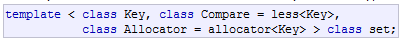接口的使用：

<1>构造一个set类型的变量

set<int> s;

<2>insert() --- 插入一个元素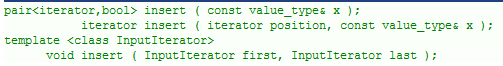- 1 第一个insert返回的是一个pair类型的变量，其包含两个成员，第一个成员的类型是该map迭代器的类型，另一个成员的类型是bool

	//构造一个int类型的set
set<int> s;

//插入几个元素
s.insert(1);
s.insert(2);
s.insert(6);

//测试insert()的返回值
pair<set<int>::iterator, bool> ret;
ret = s.insert(4);//set中还没有4这个元素，插入成功，返回插入节点的迭代器和TRUE
ret = s.insert(4);//set中已经存在了4，插入失败，返回的是元素4的迭代器和FALSE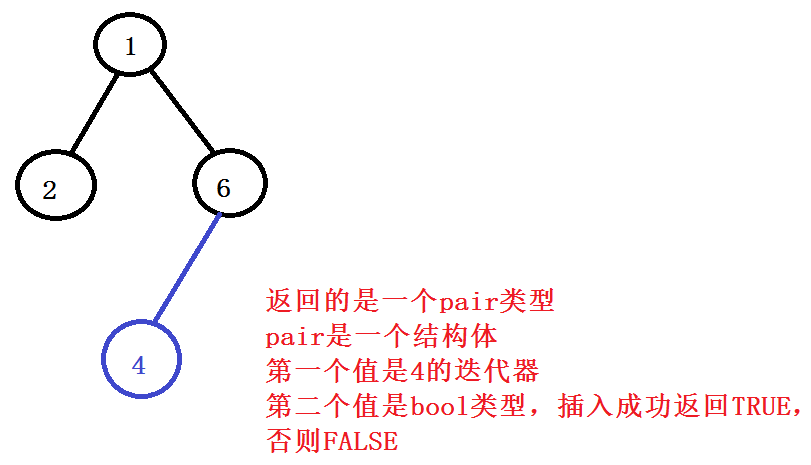- 2 该insert插入的是一段迭代器区间

	//定义一段vector，类型也是int
vector<int> v;
v.push_back(10);
v.push_back(50);
v.push_back(5);

//用insert向set s中插入该vector的全部
s.insert(v.begin(), v.end());
<3>利用迭代器访问元素

	//定义一个对应类型的迭代器，指向s的起始
set<int>::iterator it = s.begin();

//当it没有指向s的最后一个元素的后一个元素时，访问
while (it != s.end())
{
cout << *it << " ";
it++;//迭代器自增，指向下一个元素
}
cout << endl;

<4>erase() ---删除一个元素或一段区间

- 1 用erase() 删除一个元素，传入删除元素的迭代器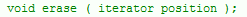- 2 用erase()删除一个元素，穿入一个key值，返回的是删除该key值节点的个数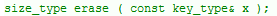- 3 删除一段迭代器区间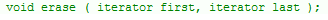//1.用find查找1这个元素，并用迭代器it获取返回值,然后进行删除
set<int>::iterator it = s.find(500);

if (it != s.end())                  //查询成功，则表示元素存在，删除该元素
s.erase(it);
else		              //若查询失败，表示不存在，对it解引用会是NULL
cout << "没有找到" << endl;

//2.直接删除一个key值
s.erase(2);

//3.删除一段迭代器区间
s.erase(s.begin(), s.end());

<5>find() --- 用来查找一个元素，若查找到，则返回该元素对应的迭代器，否则返回最后一个元素的下一个元素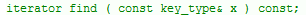<6>count()

统计一个元素的个数，在set中用于判断一个元素是否存在；因为无法插入相同的值，所以在set/map中与find的作用一样

<7>emplace()

emplace和insert都是向set中插入一个元素，但效率上看，emplace的效率要高一点

insert是先构造好，然后又经过一次拷贝构造放入set中；

map

简介：

map也是一种关联式容器，与set不同的是，它是一种 Key/Value 结构的容器；

map的几种用途：

定义：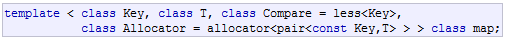接口的使用：

<1> 定义一个map

map<string,int> countMap;

<2>insert()

- 1 插入一个pair

map<string, int> m;
m.insert(pair<string, int>("hello", 0));
m.insert(pair<string, int>("world", 1));
- 2 插入一个value_type
m.insert(map<string, int>::value_type("change", 1));
- 3 利用数组下标进行插入，这里的K值需要是int
map<int, string> m;
m = "first";
m = "second";
m = "third";

<3>erase() 元素的删除

- 1 迭代器删除

map<string, int>::iterator it = m.find("hello");
if (it != m.end())
m.erase(it);
- 2 key值删除

m.erase("hello");